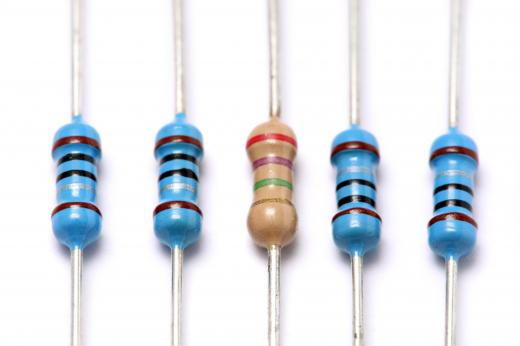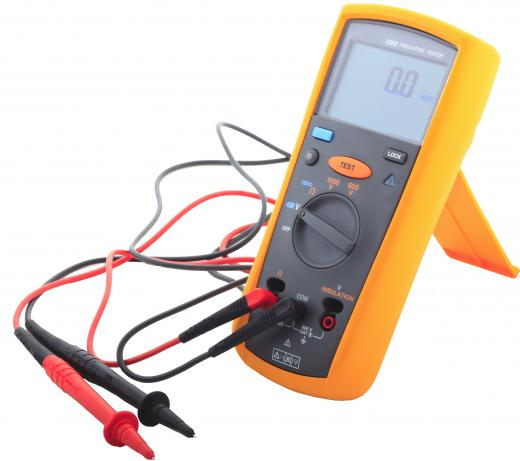Science
Fact Checked

# What is an Ohm?

Brendan McGuigan
Brendan McGuigan

An ohm is a unit used in the international system of measurements to measure resistance, represented by the Greek letter omega (Ω). By definition, it is equal to one volt creating one ampere in a device, abbreviated as V/A. An ampere, or amp, is a measure of current equal to a specific amount of force between a theoretical pair of infinite conductors in a vacuum.

Georg Ohm was a German physicist in the early 19th century. He was well-trained in both mathematics and physics, and held a number of distinguished teaching positions. In 1827, he published what is viewed by most people as his master work, Die galvanische Kette mathematisch bearbeitet, in which he laid out most of his general theory of electricity.Resistors, available in various ohm resistance values, are used to control the flow of electricity in a circuit.

Ohm is looked at by many as one of the great fathers of electricity, as his work heavily influenced all theory of the day. Ironically, it took many years for him to gain recognition in his own life. Though he strove for a professorship at a university, he received little acknowledgment for his ground-breaking work until near the end of his life. Much of this was undoubtedly due to his reliance on mathematics as a fundamental way to describe his theories on the workings of electricity. Though much cleaner and ultimately more useful than the non-mathematical physics of the day, it was difficult for much of the establishment to accept.A multimeter is a tool that can measure amps, volts, and ohms.

The equation that yields the measurement bearing his name, Ohm's Law, is a rather simple one. It is written as V/I = R, where V is the voltage of a device, I is the current, and R is the resulting resistance. It should be noted that the resistance of a real-world device is never exactly true to this law, as impurities in the conductor and the actual behavior of electrons affect the resistance. In practice, however, such discrepancies are negligible enough to be ignored, and this law may be treated as true.

An inverse measure also exists, called alternately a siemens or a mho. It may be expressed as an inverted omega, or an ohm to the negative first power. A mho may be defined as A/V, where A is amperes and V is voltage.

## You might also Likeanon360068

Ohm's Law is not a universal law and does not apply to most electrical conductors or devices. For Ohm's Law to apply, the ratio of voltage to current must remain constant for variations in voltage and this is rarely the case.

Those materials that do obey Ohm's Law are called 'linear' or 'ohmic', whereas the majority of materials that don't obey Ohm's Law are called 'non-linear' or 'non-ohmic'. The equation, resistance equals voltage divided by resistance is not derived from Ohm's Law, but from the definition of the ohm, and this equation applies to all materials.

anon351455

The relationship between resistance, current and voltage is called ohm's law.

anon229468

The original volt was based on the EMF from a standard cell (the LeClanche cell, if I remember correctly). The original ohm was based on the resistance that would produce a certain amount of heat when connected to a standard volt. The current that flowed when 1 volt was applied to 1 ohm was called an ampere. Then things got turned around. The ampere was redefined as the current that causes a specific force between two conductors, the ohm was redefined as the resistance dissipating a certain amount of heat when the newly defined ampere flowed, and the volt became the potential difference across that resistance when one ampere flowed. One ampere flowing for 1 second carried a charge of 1 coulomb, which was later determined to be equivalent to (approximately) 6x10^18 electrons or protons.

Now, the ampere is set to be redefined in terms of the charge it carries, and everything will be redefined in terms of the charge on an electron which was based on the ampere in the first place! So as with the clumsy metric system, which is based on the number of fingers we have, and the second which was based on who knows what, yet again a standard will be reverse-engineered to make the bits fit.

anon35088

exactly. you talk about varistors. they still follow ohm's law. the resistance may change, but when it does, the ratio between the voltage and current also changes. it would be violating ohm's law if one changes *but not the other.* since they both change in proportion, it is still true.

anon22998

The post by anon21021 should be revised to eliminate the statement that ohms law is violated. It isn't. The fact that resistance is changing in a device does not mean ohms law is wrong. It only means there is a different and dynamic operating point, like described for the light bulb. -Ed Miska

anon21021

There are devices that violate ohm's law; they are, consequently, called "non-ohmic." Such devices include varistors, used in surge protectors, and memristors.

anon845

The fact is Ohm's Law is a law and laws (in scientific terms) are never broken. The only reason some some 'devices' don't SEEM to be obeying the law is due to heat. In a light bulb it appears that it isn't obeying the law yet its the fact that over time it heats up and the atoms move.